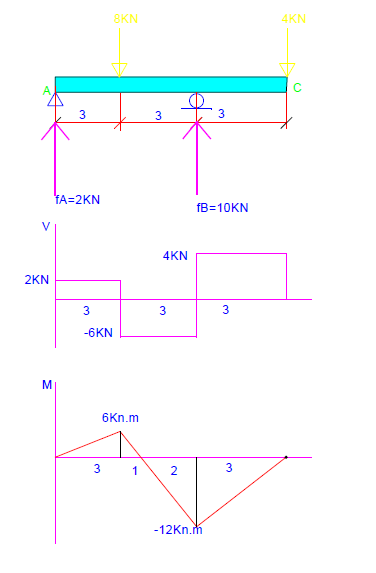### Conjugate beam Problem no:2 (beam with pin and internal roller)

Problem no:2, Determine the slope and displacement for the following beam at point C (E=200Gpa, I=70*10^6 mm2)?Figure 1

At point A, the pin support will not change, while the roller support at B will change to an internal pin for the conjugate beam. Also, the free end at C will become fixed support in the conjugate beam. So the conjugate beam will be as shown in figure no:2.
Figure 2

We need to determine the M/EI diagram for the real beam, which will be the conjugate beam load. Using the equilibrium equations, we will determine the reaction at point A and point B.
Now we can draw the shear diagram for the beam. The moment will be the area of the shear diagram. The shear and moment diagram for the real beam will be as shown in figure no:4. The M/EI will be the load for the conjugate beam. So the conjugate beam will be loaded as shown in figure no:5.Figure 4

Figure 5

To determine the slope and displacement at C, we need to determine the shear and moment at C for the conjugate beam. To simplify the solution, we will divide the beam into two sections, and we will start with section A. as we know, the internal hinge carries the only force and no moment.

Section A

Now section B